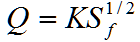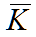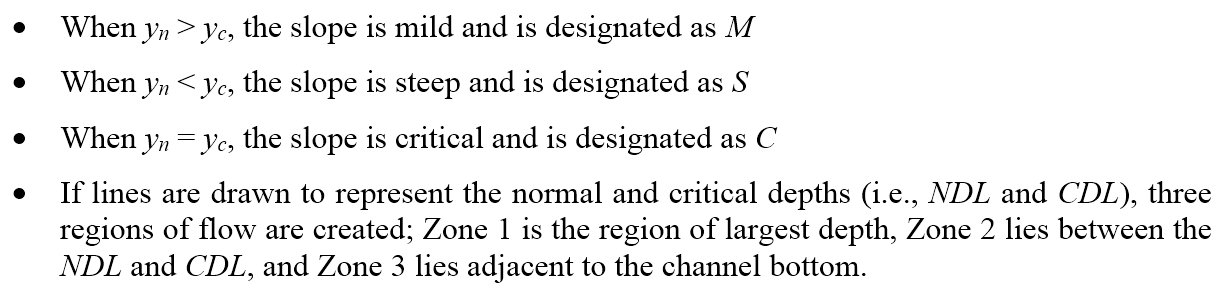hydraulics

# Gradually Varied Flow in Innovyze H2OCalc

Discussions in the previous sections have focused on uniform flow hydraulics where flow depth y and flow velocity V remain constant along the channel. In this section, we consider gradually varied flow, which is a form of steady nonuniform flow characterized by gradual variations in flow depth and velocity and a free surface that always remains smooth (no abrupt changes).The following equation represents the general energy equation for gradually varied flow (see the above figure), for a reach ofin length

The friction slope Sf can be expressed using Manning’s equationwhere K is defined as the conveyance factorin SI units orin English units.

Hencewhereis average of the upstream and the downstream conveyance factors.

Water surface profile for a channel can be generated by solving Equation (13). The channel would be divided into short reaches and computation for water surface elevation would be progressed from one end of the reach to the other end. The two most commonly used techniques for water surface profile computation are the direct step method and the standard step method. In the direct step method, depth at the other end of the reach is assumed and the length () of the reach would be evaluated based on known depth and velocity information at one end of the reach. In the contrary, the computation procedure of the standard step method involves determination of the depth based on predetermined reach length ().

Equation 13 can be used to derive the following two alternative forms of the gradually-varied flow equations.

Equation 14 can be used to qualitatively evaluate gradually varied flow profiles on various channel slopes. The evaluation and subsequent classification of shapes is an important preliminary step to computing associated water surface profiles since it yields information about the location of hydraulic controls, the direction of computation (i.e., upstream for subcritical flow or downstream for supercritical flow), and whether depth is increasing or decreasing. Classification of profiles begins by establishing criteria upon which the water surface depends, namely slope of the channel and depth of flow relative to the critical and normal depths (i.e., yc and yn). The criteria are as follows:These criteria can be used to define the water surface profiles shown in the following figure. For example, an M1 profile exists when y > yn > yc. Under these conditions, Fr < 1 since y> yc, and Sf < So since y > yn. Thus, the sign of the numerator and denominator in Equation 14 are both positive, and dy/dx must be positive. The implication is that depth will increase with x, typically referred to as a backwater curve. Flow will also approach the normal depth upstream. Alternatively, for an M2 profile, yn > y > yc, so that the sign of dy/dxmust be negative. In this case, depth decreases with x, which represents a drawdown curve. Similar analyses can be made for other profiles. Note that horizontal and adverse slopes are unique cases in that the normal depth does not exist for either case. In addition, certain theoretical aspects of some profiles do not correlate well with realistic, physical behavior. For example, complete development of an M3 profile is unlikely; upstream flow cannot be zero, and the downstream end of the profile will likely be overcome by a hydraulic jump.